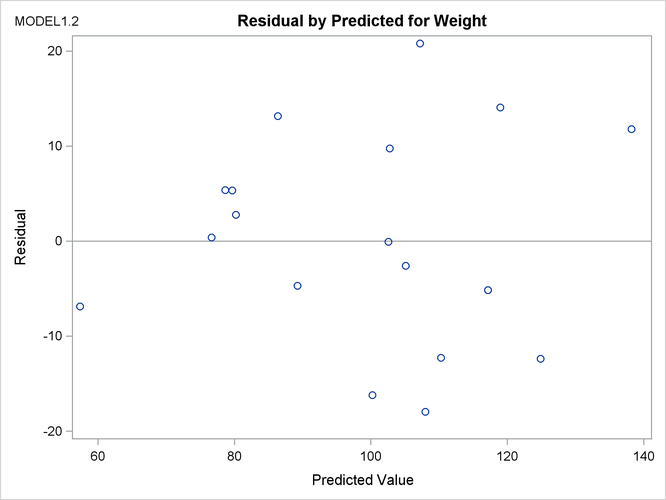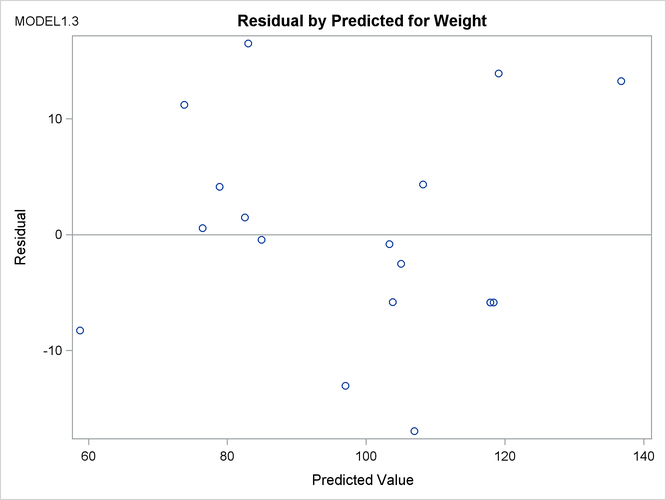### Interactive Analysis

PROC REG enables you to change interactively both the model and the data used to compute the model, and to produce and highlight scatter plots. See the section Using PROC REG Interactively for an overview of interactive analysis that uses PROC REG. The following statements can be used interactively (without reinvoking PROC REG): ADD, DELETE, MODEL, MTEST, OUTPUT, PAINT, PLOT, PRINT, REFIT, RESTRICT, REWEIGHT, and TEST. All interactive features are disabled if there is a BY statement.

The ADD, DELETE, and REWEIGHT statements can be used to modify the current MODEL. Every use of an ADD, DELETE, or REWEIGHT statement causes the model label to be modified by attaching an additional number to it. This number is the cumulative total of the number of ADD, DELETE, or REWEIGHT statements following the current MODEL statement.

A more detailed explanation of changing the data used to compute the model is given in the section Reweighting Observations in an Analysis.

The following statements illustrate the usefulness of the interactive features. First, the full regression model is fit to the `Sashelp.Class` data, and Figure 79.25 is produced.

```ods graphics on;

proc reg data=sashelp.Class plots(modelLabel only)=ResidualByPredicted;
model Weight=Age Height;
run;
```

Figure 79.25: Interactive Analysis: Full Model

The REG Procedure
Model: MODEL1
Dependent Variable: Weight

Analysis of Variance
Source DF Sum of
Squares
Mean
Square
F Value Pr > F
Model 2 7215.63710 3607.81855 27.23 <.0001
Error 16 2120.09974 132.50623
Corrected Total 18 9335.73684

 Root MSE R-Square 11.5111 0.7729 100.026 0.7445 11.5081

Parameter Estimates
Variable DF Parameter
Estimate
Standard
Error
t Value Pr > |t|
Intercept 1 -141.22376 33.38309 -4.23 0.0006
Age 1 1.27839 3.11010 0.41 0.6865
Height 1 3.59703 0.90546 3.97 0.0011

Next, the regression model is reduced by the following statements, and Figure 79.26 is produced.

```   delete age;
print;
run;
```

Figure 79.26: Interactive Analysis: Reduced Model

Analysis of Variance
Source DF Sum of
Squares
Mean
Square
F Value Pr > F
Model 1 7193.24912 7193.24912 57.08 <.0001
Error 17 2142.48772 126.02869
Corrected Total 18 9335.73684

 Root MSE R-Square 11.2263 0.7705 100.026 0.7570 11.2233

Parameter Estimates
Variable DF Parameter
Estimate
Standard
Error
t Value Pr > |t|
Intercept 1 -143.02692 32.27459 -4.43 0.0004
Height 1 3.89903 0.51609 7.55 <.0001

Note that the MODEL label has been changed from MODEL1 to MODEL1.1, since the original MODEL has been changed by the delete statement.

When ODS Graphics is enabled, updated plots are produced whenever a PRINT statement is used. The option

```plots(modelLabel only)=ResidualByPredicted
```

in the PROC REG statement specifies that the only plot produced is a scatter plot of residuals by predicted values. The MODELLABEL option specifies that the current model label is added to the plot.

The following statements generate a scatter plot of the residuals against the predicted values from the full model. Figure 79.27 is produced, and the scatter plot shows a possible outlier.

```   add age;
print;
run;
```

Figure 79.27: Interactive Analysis: Scatter PlotThe following statements delete the observation with the largest residual, refit the regression model, and produce a scatter plot of residuals against predicted values for the refitted model. Figure 79.28 shows the new scatter plot.

```   reweight r.>20;
print;
run;

ods graphics off;
```

Figure 79.28: Interactive Analysis: Scatter Plot